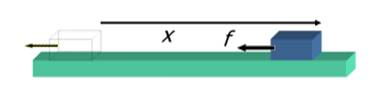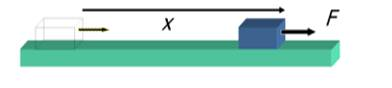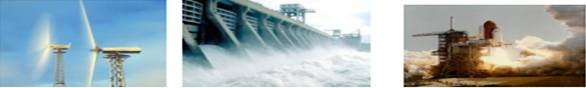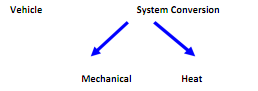### Work and Energy - Revision Notes

CBSE Class 9 Science

Revision Notes
CHAPTER – 11
Work & Energy

1. Work Done By A Constant Force

• Work is a scalar quantity equal to the product of the displacement x and the component of the force Fx in the direction of the displacement..
• Work is defined as a force acting upon an object to cause a displacement
• Mathematically, work can be expressed by the following equation.
• where F - force, d - displacement, and the angle (theta) is defined as the angle between the force and the displacement vector
• Three things are necessary for the performance of work:
• There must be an applied force F.
• There must be a displacement x.
• The force must have a component along the displacement

Negative WorkThe friction force f opposes the displacement

Positive WorkForce F contributes to displacement x.

2. Energy And Its Forms

The metric system unit of energy is the joule (J), after James Joule.

• Mechanical energy is the energy which is possessed by an object due to its motion or its stored energy of position

Forms of Energy

• Kinetic energy : is the energy of motion

Energy which a body possesses because of its motion, which occurs anywhere from an atomic level to that of a whole organismExamples of Kinetic Energy: This is not an all-inclusive list.

• Electrical – The movement of atoms
• Electromagnetic or Radiant – The movement of waves
• Thermal or Heat – The movement of molecules
• Motion – The movement of objects
• Sound – The movement through waves

Engineers generally refer to thermal/heat energy as “internal energy” and use “kinetic energy” strictly in reference to motion. Potential Energy (Stored energy or gravitational energy)

• The capacity to do work by virtue of position or configuration
• an object can store energy as the result of its position or elastic source
• Potential Energy is maximum at the maximum HEIGHT

Energy transformation involves the conversion of one form of energy into another form. Examples of energy transformation include:

• Chemical – Food is consumed and converted into motion for playing sports or taking a test.

• Radiant – Sunlight is consumed by plants and converted into energy for growth.

• Electrical – Energy transferred to an oven is converted to thermal energy for heating our food.

Now you know the basic forms of energy. The next question is “What are the energy sources?”

There are renewable and nonrenewable sources of energy. A renewable energy source is a form of energy that is constantly and rapidly replenished by natural processes.

Examples of renewable energy sources include:

• Biomass – The use of a living or once living organism as fuel

• Hydropower – The energy produced from the movement of water

• Geothermal – The use of heat from within the Earth or from the atmosphere near oceans to warm houses or other buildings

• Wind – The use of wind to generate electricity

Solar – The use of the sun as a source of heat; for instance, to heat a room within a house, etc.

Energy Conversion

Fossil fuels Chemical → Heat → Mechanical → Electrical

Solar cells Sunlight → Electrical

Wind turbines Kinetic → Mechanical → Electrical

Hydroelectric Gravitational potential → Mechanical → Electrical

Nuclear Nuclear → Heat → Mechanical → Electrical3. Potential energy of an object at a height

An object increases its energy when raised through a height.

The potential energy of an object at a height depends on the ground level or the zero level

4. Law Of Conservation Of Energy

The principle of Conservation of Mechanical Energy

The total mechanical energy $\left(\text{E = KE}+\text{PE}\right)$ of an object remains constant as the object moves, provided that the net work done by external non-conservative forces is zero, ${\text{W}}_{nc}=0\text{J}$

Total mechanical energy: the sum of kinetic energy and gravitational potential energy

E=KE+PE

${W}_{nc}=\left(K{E}_{f}-K{E}_{0}\right)+\left(P{E}_{f}-P{E}_{0}\right)$

${W}_{nc}=\left(K{E}_{f}+P{E}_{f}\right)-\left(K{E}_{0}+P{E}_{0}\right)$

${W}_{nc}={E}_{f}-{E}_{0}$

${E}_{f}=-K{E}_{f}+P{E}_{f}$

$E=K{E}_{0}+P{E}_{0}$

5. Rate of Doing Work & Commercial Unit Of Energy POWER

Rate at which work is performed or energy is expended $P=\frac{W}{t}$

Watt is the base unit of Power .One watt is equal to 1 joule of work per second

Types of Power

• Electrical Power

Uses electrical energy to do work

• Mechanical Power

Uses mechanical energy to do work (linear, rotary)

• Fluid Power

Uses energy transferred by liquids (hydraulic) and gases (pneumatic)

• Power is the rate that we use energy.

• Power = Work or Energy / Time

•

• The unit joule is too small .The bigger unit of energy called kilowatt hour (kW h)

1 kW h is the energy used in one hour

at the rate of